Download Presentation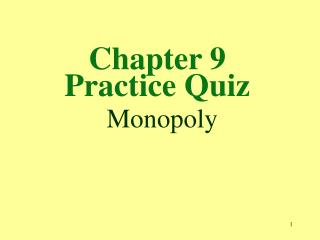Chapter 9 Practice Quiz Monopoly

# Chapter 9 Practice Quiz Monopoly - PowerPoint PPT Presentation

Chapter 9 Practice Quiz Monopoly. 1. A monopolist always faces a demand curve that is a. perfectly inelastic. b. perfectly elastic. c. unit elastic. d. the same as the market demand curve.I am the owner, or an agent authorized to act on behalf of the owner, of the copyrighted work described.
Download Presentation## Chapter 9 Practice Quiz Monopoly

An Image/Link below is provided (as is) to download presentation

Download Policy: Content on the Website is provided to you AS IS for your information and personal use and may not be sold / licensed / shared on other websites without getting consent from its author.While downloading, if for some reason you are not able to download a presentation, the publisher may have deleted the file from their server.

- - - - - - - - - - - - - - - - - - - - - - - - - - E N D - - - - - - - - - - - - - - - - - - - - - - - - - -
Presentation Transcript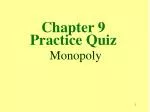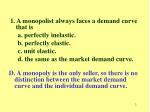1. A monopolist always faces a demand curve that is

a. perfectly inelastic.

b. perfectly elastic.

c. unit elastic.

d. the same as the market demand curve.

D. A monopoly is the only seller, so there is no distinction between the market demand curve and the individual demand curve.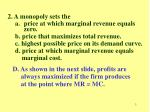2. A monopoly sets the
• price at which marginal revenue equals zero.

b. price that maximizes total revenue.

c. highest possible price on its demand curve.

d. price at which marginal revenue equals

marginal cost.

D. As shown in the next slide, profits are

always maximized if the firm produces

at the point where MR = MC.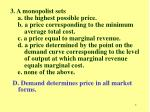3. A monopolist sets

a. the highest possible price.

b. a price corresponding to the minimum

average total cost.

c. a price equal to marginal revenue.

d. a price determined by the point on the

demand curve corresponding to the level

of output at which marginal revenue

equals marginal cost.

e. none of the above.

D. Demand determines price in all market forms.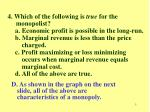4. Which of the following is true for the

monopolist?

a. Economic profit is possible in the long-run.

b. Marginal revenue is less than the price

charged.

c. Profit maximizing or loss minimizing

occurs when marginal revenue equals

marginal cost.

d. All of the above are true.

D. As shown in the graph on the next slide, all of the above are characteristics of a monopoly.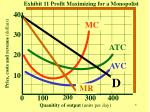.

Exhibit 11 Profit Maximizing for a Monopolist

40

MC

30

Price, costs and revenue (dollars)

ATC

20

AVC

10

D

MR

100

200

300

400

0

Quanitity of output (units per day)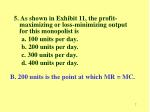5. As shown in Exhibit 11, the profit-maximizing or loss-minimizing output for this monopolist is

a. 100 units per day.

b. 200 units per day.

c. 300 units per day.

d. 400 units per day.

B. 200 units is the point at which MR = MC.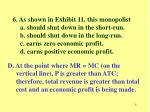6. As shown in Exhibit 11, this monopolist

a. should shut down in the short-run.

b. should shut down in the long-run.

c. earns zero economic profit.

d. earns positive economic profit.

D. At the point where MR = MC (on the

vertical line), P is greater than ATC;

therefore, total revenue is greater than total

cost and an economic profit is being made.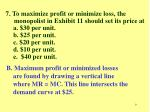7. To maximize profit or minimize loss, the

monopolist in Exhibit 11 should set its price at

a. \$30 per unit.

b. \$25 per unit.

c. \$20 per unit.

d. \$10 per unit.

e. \$40 per unit.

B. Maximum profit or minimized losses

are found by drawing a vertical line

where MR = MC. This line intersects the

demand curve at \$25.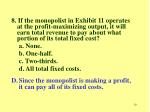8. If the monopolist in Exhibit 11 operates at the profit-maximizing output, it will earn total revenue to pay about what portion of its total fixed cost?

a. None.

b. One-half.

c. Two-thirds.

d. All total fixed costs.

• Since the monopolist is making a profit, it can pay all of its fixed costs.9. For a monopolist to practice effective price

discrimination, one necessary condition is

• identical demand curves among groups of buyers.

b. differences in the price elasticity of demand among groups of buyers.

c. a homogeneous product.

d. none of the above.

B. Price discrimination takes place when a monopolist is faced with buyers that are widely different; therefore, the buyers elasticity of demand for the product will be different.10. What is the act of buying a commodity at a

lower price and selling it at a higher price?

b. Discounting.

c. Tariffing.

d. Arbitrage.

D. The practice of earning a profit by

buying a good at a low price and

reselling the good at a higher price.11. Under both perfect competition and

monopoly, a firm

a. is a price taker.

b. is a price maker.

c. will shut down in the short run if price falls

short of average total cost.

d. always earns a pure economic profit.

e. sets marginal cost equal to marginal

revenue.

E. The profit maximizing output for any

firm is where MR = MC.12. At any point where a monopolist’s

marginal revenue is positive, the

downward-sloping straight-line demand

curve is

a. perfectly elastic.

b. elastic, but not perfectly elastic.

c. unitary elastic.

d. inelastic.

B. When marginal revenue is zero, the demand curve is unitary elastic, and it is inelastic when marginal revenue is negative. Demand is perfectly elastic in perfect competition.intersection of the marginal cost and marginal revenue

curves. If this price is between its average variable cost and

average total cost curves, the firm will

a. earn an economic profit.

b. stay in operation in the short-run, but shut down in the

long run if demand remains the same.

c. shut down.

d. none of the above.

B. If the firm can cover its average variable cost, it is paying for the cost of operating. In the long run, demand could increase and the firm earns economic profit.14. In contrast to a perfectly competitive firm,

a monopolist operates in the long run at a

quantity of output at which

a. P = MC.

b. MR = MC.

c. P = ATC.

d. P > MR.

D. The perfectly competitive firm’s demand curve is perfectly elastic; therefore, the firms operates where P = MR. For a monopolist, the MR curve is downward sloping and below the price.15. The monopolist, unlike the perfectly

competitive firm, can continue to earn an

economic profit in the long run because of

a. collusive agreements with competitors.